# Tail Recursive nth Fibonacci Number

When a function is called, it gets it’s own stack to store the parameters, and it’s local variables. So, an implementation of recursive function that stores a local variable and waits for the values returned from another recursive call to the function and so on would require stack to store the results. Let’s see an example of a recursive function implemented in Standard ML (SML), a general-purpose functional programming language,

``````fun factorial n =
if n = 0 then 1
else n * (factorial (n - 1))``````

I will base my writing for a functional programming language and will use SML as my language of choice. Python, however, does not optimize tail recursive calls by default. And we are still bounded by default maximum recursion depth of 1000 (we can manually increase this limit). However, I will also write python equivalent code.

Python equivalent code
``````
def factorial(n):
if n == 0:
return 1
else:
return n * factorial(n - 1)
``````

The above code that calculates the factorial of a given number n, requires stack to store all values of n unless base condition is met. So, if n is really big, we can see how stack space grows linearly with n. One way of solving this is to write the above recursive function using tail recursion. We call a function tail recursive if no computation happens after a recursive call. In our case the above factorial() function would look like

``````(* HELPER FUNCTION TO CALCULATE FACTORIAL *)
fun calculate_fact r n =
if n = 0 then r
else calculate_fact (r * n) (n - 1)

(* MAIN FUNCTION *)
fun factorial n = calculate_fact 1 n ``````
Python equivalent code
``````def factorial(n, r=1):
if n == 0:
return r
else:
return factorial(n - 1, r * n)
``````

In this above program, we do not need to save the local variables as we did in the first program because they are not referenced after the recursive call. Hence, compiler can optimize the tail recursion and there’s no use of saving the current function’s stack frame. One way of thinking tail recursion would be as an iteration in disguise as used in imperative language. For example, this above tail recursion could be coded as

``````def factorial(n):
r = 1
while n != 0:
r = r * n
n = n - 1
return r``````

One thing to note is, ideally we would want tail recursion not to be bounded by maximum recursion depth. However, python has a default recursion depth of 1000 and we cannot go past this limit without increasing it. But functional languages do not have this limit.

Now let’s look into these concept in case of Fibonacci sequence.

Fibonacci sequence is generated by Fibonacci numbers where each number is the sum of two preceding ones, starting from 0 and 1. The sequence goes like 0, 1, 1, 2, 3, 5, 8, 13, 21, 34, 55, 89, 144, 233, ….. and so on. So,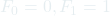and for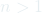,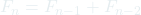. Some implementation ignores 0 and,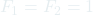is taken as starting of the sequence. We will use the convention that 1 is the first element in the sequence.

We can see that numbers can get really big quickly. Hence, to generate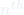Fibonacci number, we might want to evaluate the performance of our algorithm even if it gives correct results for smaller value of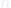. Let’s see some approaches, their pitfalls, and improvements we could make.

A straight forward solution to calculateFibonacci number is to recursively calculate sum of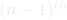and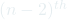Fibonacci numbers.

``````fun fibonacci n =
if n = 0 then 0
else if n = 1 then 1
else (fibonacci (n - 1)) + (fibonacci (n - 2));``````
Python equivalent code
``````def fibonacci(n):
if n == 0:
return 0
elif n == 1:
return 1
else:
return fibonacci(n - 1) + fibonacci (n - 2)``````

Running this python equivalent function fibonacci(35) took 4.0823 seconds in my computer.
If we analyze this function, we can see two main drawbacks of this implementation,

1. It is not tail recursive and so requires stack space to run.
2. It repeatedly calls the fibonacci function on smaller numbers i.e. recomputing previously computed results. For example,
fibonacci (7)
=> fibonacci (6) + fibonacci (5)
=> fibonacci (5) + fibonacci (4) + fibonacci (5)
=> fibonacci (4) + fibonacci (3) + fibonacci (4) + fibonacci (5) … and so on

Time complexity: Exponential
Space complexity: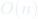(stack usage)

Let’s write this fibonacci function using tail recursion.

``````(* HELPER FUNCTION TO CALCULATE FIBONACCI NUMBER *)
fun fib_calculate prev curr n =
if n = 0 orelse n = 1 then curr
else fib_calculate curr (prev + curr) (n - 1);

(* MAIN FUNCTION TO ABSTRACT prev AND curr VALUES *)
fun fibonacci n = fib_calculate 0 1 n;``````
Python equivalent code
``````def fibonacci(n, prev=0, curr=1):
if n == 0 or n == 1:
return curr
else:
return fibonacci(n - 1, curr, prev + curr)``````

Running this function fibonacci(35) took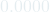seconds in my computer. Here, we do not recompute previously computed results and also, stack space is constant and does not grow with the size of input n. For this implementation,

Time complexity:Space complexity: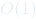So, we reduced the exponential time complexity to linear and stack space complexity from linear to constant by using tail recursion and preventing duplicate computations.

Fun Fact: There exists mathematical formula we can calculateFibonacci number with constant time and space complexity. It’s called Binet’s Formula.